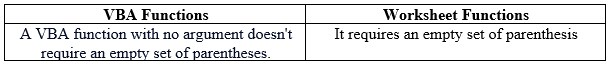# VBA Worksheet FunctionArticle byExcelMojo Team## What are Excel VBA Worksheet Functions?

Worksheets are sheets we work on in Excel in the form of rows and columns. Worksheet Function is the Application object’s method and allows you to access Excel functions in VBA. To understand the use of the Worksheet function in VBA, let us see a simple example. We must select a sheet in the worksheet using the VBA code and the worksheet function “Select.”

Open a worksheet in Excel, there are Sheet1, Sheet2, etc. Now, open a new module in the VB editor and write the below code:

Code:

Sub VBAWSExample1()
Worksheets(“Sheet3”).Select
End Sub

Run the code using F5 or click the Run option in the VB ribbon. You can see that Sheet3 is selected in the worksheet, as shown below.

###### Key Takeaways
• VBA Worksheet Functions is used to access a particular worksheet in an Excel workbook for working upon.
• You can use the WorksheetFunction property to access worksheet functions in a macro (VBA).
• It offers access to several (not all) standard Excel worksheet functions.
• We use different WorksheetFunction object to perform different tasks in VBA.
• To avoid an error, write the correct names of sheets or the specified cell.

### How to use Worksheet Functions in VBA?

Let us take a simple example of the worksheet function “Name.” We can change the sheet’s name by using this function in VBA. Please follow the below steps to know the use of the Worksheet function in VBA:

Step 1: To open the VBA options, go to the “Developer” tab and click on the “Visual Basic” option.

Step 2: After clicking on the “Visual Basic” option, “Microsoft Visual Basic for Applications” windows will open.

Step 3: Click “Insert” to open a new “Module,” and you are ready to type your VBA code in the VBA editor.

Step 4: Start writing the code with the sub-procedure.

Step 5: Write the Worksheet function for Sheet2 and specify the name to be given to it as shown below:

Sub VBAWSEx()
Worksheets(“Sheet2”).Name = “VBA WS Example 2”
End Sub

Step 6: Run the code using the VB ribbon’s F5 or the “Run” option. The result is shown below.

### Examples

Let us see a few examples of how to use the Worksheet function in VBA.

#### Example #1

To use the SUM Worksheet Function.

This Worksheet function adds the desired data set within rows and columns. Let us see how we can sum up the monthly sales of products using the SUM worksheet function.

Step 1: Write the below data in an Excel sheet and open the VB editor to write the code.

Step 2: Start writing the code with a sub-procedure, as shown below.

Step 3: Mention the cell address where we want the sum and define the range of cells to which the SUM function is to be applied.

Code:

Sub VBAWSExample1()
Range(“B9”).Value = WorksheetFunction.Sum(Range(“B2:B8”))
End Sub

Step 4: Run the code using F5 or using the Run option in the VB ribbon to see the sum of the total sales in cell B9, as shown below.

#### Example #2

Use of VLOOKUP as a Worksheet Function

In this example, we will see the use of the VLOOKUP function to find the desired value in the worksheet using VBA code.

Step 1: Suppose we have the salary of 3 employees and wish to find the salary of one employee, let’s say “Henry.”

Step 2: We start with the sub procedure VBAEX2() and declare myResult as a Variant.

Step 3: Now, define the condition for myResult using the worksheet function VLOOKUP for finding “Henry” in the specified cell range, as shown below.

Sub VBAEX2()
Dim myResult As Variant
myResult = Application.WorksheetFunction.VLookup(“HENRY”, Range(“A2:B4”), 2, False)
End Sub

Step 4: Assign the message box to see the result as shown below.

Step 5: Run the code using F5 or the Run option in the VB editor and we can see the result in the message box.

### Important Things To Note

• We must use either WorksheetFunction directly or as Application to use the Worksheet function in VBA.WorksheetFunction.
• The Worksheet function is a method of the Application object.
• The worksheet function COUNTA in VBA is used to count the number of cells in the worksheet, and COUNTIF in VBA is used to count the number of cells within a range that meet the specified criteria.
• We do not have access to all Worksheet functions in VBA. Hence, we use Application.WorksheetFunction before the function you wish to call.

1. Why is VBA Worksheet Function not working?

In Microsoft Visual Basic for Applications, only limited worksheet functions are supported as methods of the Application object. To work with a worksheet function in VBA, we must use WorksheetFunction” or “Application.WorksheetFunction.”

2. What is the difference between the VBA function and the worksheet function?3. What is the difference between the application and worksheet function in VBA?

The difference between WorksheetFunction and Application is that WorksheetFunction provides intellisence but can cause random resolution problems (the function referenced might not be found or resolved.)